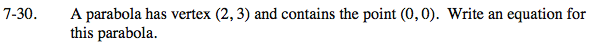Home > INT3 > Chapter 7 > Lesson 7.1.2 > Problem7-30

7-30.

A parabola has vertex (2, 3) and contains the point (0, 0). Write an equation for this parabola. Homework Help ✎For a vertically-oriented parabola, use the equation y = a(xh)2 + k where (h, k) = (2, 3) and (x, y) = (0, 0).
For a "sleeping parabola" use the equation x = a(yk)2 + h.

0 = a(0 − 2)2 + 3

−3 = a(4)

$-\frac{3}{4}=a$

$y=-\frac{3}{4}(x-2)^2+3$